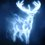# How to find which day of the week?

There are many methods available for determining the day of the week given the day , month and year on that day . However , most methods are suitable for mental calculations . The following formula , which is for the Gregorian Calendar only , may be more convenient for computer programming .

W = ( k + floor function of (2.6 m - 0.2) - 2 *C + Y + floor function of (Y/4) + floor function of (C/4) )mod 7.

where
- floor function returns the largest integer < = n
- k is day (1 to 31)
- m is month ( 1= March ( it's Gregorian remember) , .........10 = December , 11 = Jan etc)
- C is century ( like for 2016 it is 20 and not 21 ( blimey !))
- Y is year ( 2016 has Y= 16 except 15 for Jan to Feb ( Phew !))
W is weekday ( 0 = Sunday ..............6 = Saturday )

For the pochemuchka , here the century and 400 year corrections are inbuilt into the formula . For some years , the W results into a negative number which is to be corrected by adding 7 to W .Note by Raven Herd
5 years, 2 months ago

This discussion board is a place to discuss our Daily Challenges and the math and science related to those challenges. Explanations are more than just a solution — they should explain the steps and thinking strategies that you used to obtain the solution. Comments should further the discussion of math and science.

When posting on Brilliant:

• Use the emojis to react to an explanation, whether you're congratulating a job well done , or just really confused .
• Ask specific questions about the challenge or the steps in somebody's explanation. Well-posed questions can add a lot to the discussion, but posting "I don't understand!" doesn't help anyone.
• Try to contribute something new to the discussion, whether it is an extension, generalization or other idea related to the challenge.
• Stay on topic — we're all here to learn more about math and science, not to hear about your favorite get-rich-quick scheme or current world events.

MarkdownAppears as
*italics* or _italics_ italics
**bold** or __bold__ bold
- bulleted- list
• bulleted
• list
1. numbered2. list
1. numbered
2. list
Note: you must add a full line of space before and after lists for them to show up correctly
paragraph 1paragraph 2

paragraph 1

paragraph 2

[example link](https://brilliant.org)example link
> This is a quote
This is a quote
    # I indented these lines
# 4 spaces, and now they show
# up as a code block.

print "hello world"
# I indented these lines
# 4 spaces, and now they show
# up as a code block.

print "hello world"
MathAppears as
Remember to wrap math in $$ ... $$ or $ ... $ to ensure proper formatting.
2 \times 3 $2 \times 3$
2^{34} $2^{34}$
a_{i-1} $a_{i-1}$
\frac{2}{3} $\frac{2}{3}$
\sqrt{2} $\sqrt{2}$
\sum_{i=1}^3 $\sum_{i=1}^3$
\sin \theta $\sin \theta$
\boxed{123} $\boxed{123}$

## Comments

There are no comments in this discussion.

×

Problem Loading...

Note Loading...

Set Loading...